## Generating ABCD state-space matrices

The Linearize command generates the ABCD state-space matrices from a nonlinear system by numerically evaluating the matrix perturbation equations at the time the simulation was halted and Embed was invoked. For a general multiple-input-multiple-output (MIMO) system, the ABCD state-space matrices contain the following coefficients to the vector equations: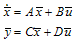where:

A = system matrix

B = input gain matrix

C = output gain matrix

D = direct link gain matrix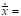state vector derivative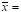state vector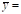output vector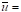input vector

The Linearize command uses a central differencing scheme to approximate the partial derivative terms at the specified operating point. The four resulting matrices can be displayed directly on the screen or saved in an M file.

To generate and display ABCD state-space matrices

1.    Prepare the system for linearization.

2.    Choose Analyze > Linearize.

3.    In the System Linearization dialog box under Output Selection, activate Linearize To Screen Display.

4.    Click OK, or press ENTER.

Four dialog boxes appear in succession showing each matrix.

5.    Click OK at the bottom of the dialog box, or press ENTER, to close the current matrix dialog box and open the next one.

To generate and write ABCD state-space matrices to an M file

1.    Prepare the system for linearization.

2.    Choose Analyze > Linearize.

3.    In the System Linearization dialog box under Output Selection, activate Linearize To MatLab File.

4.    In the Result File box, specify the name of the M file to which the matrices are to be written. When you select this parameter, enter the complete pathname for the M file. The following default specifications are used for the parts of the pathname that you omit:

 If you omit This is used The disk drive C: The directory name The directory from which you opened Embed The file name VABCD The file extension M

5.    Click OK, or press ENTER.

The Linearize command writes the matrices to the specified file that can be read into MATLAB or Altair Compose.

1.    Prepare the system for linearization.

2.    Choose Analyze > Linearize.

3.    In the System Linearization dialog box, click Select Input/Output Points.

The pointer becomes a crossbar.

4.    To select an input reference point, click over the output connector tab on the block that produces the signal with which you want to linearize.

The output connector tab changes color. If the connector tab you try to select is invalid, you hear a beep.

5.    To select an output reference point, click over the input connector tab on the block that consumes the signal against which you want to linearize.

The input connector tab changes color. If the connector tab you try to select is invalid, Embed beeps.

6.    Click over empty screen, at least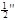from any block to exit this mode.